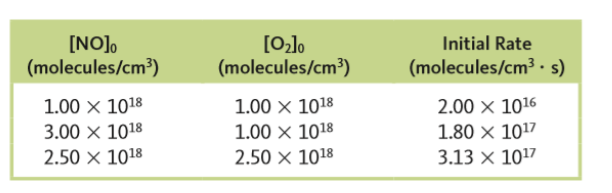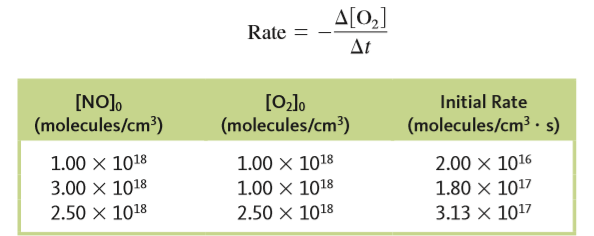# Problem: The reaction2NO (g) + O2 (g) → 2NO2 (g)was studied, and the following data were obtained whereWhat would be the initial rate for an experiment where [NO] 0 = 6.21x 1018 molecules/cm3 and [O2]0 =  7.36 x 1018 molecules/cm3?

###### FREE Expert Solution

Recall that the rate law only focuses on the reactant concentrations and has a general form of:

k = rate constant
A & B = reactants
x & y = reactant orders

Step 1. Calculate order with respect to NO:

x = order

$\frac{\mathbf{1}\mathbf{.}\mathbf{80}\mathbf{×}{\mathbf{10}}^{\mathbf{17}}}{\mathbf{2}\mathbf{.}\mathbf{00}\mathbf{×}{\mathbf{10}}^{\mathbf{16}}}\mathbf{=}{\left[\frac{3.00×{10}^{18}}{1.00×{10}^{18}}\right]}^{\mathbf{x}}\phantom{\rule{0ex}{0ex}}\mathbf{9}\mathbf{=}{\left[3\right]}^{\mathbf{x}}$

3 raised to the 2nd power = 9

x = 2 → 2nd order with respect to NO

Step 2. Calculate order with respect to O2:

y = order

since the numerator and the denominator is raised to the same power, you can simplify the equation to:

0.4 raised to the power of 1 = 0.4

y = 1 → 1st order with respect to O2Step 3Calculate the value of the rate constant

Go back to the given data. You can use any experimental data to calculate for k, but let’s use the 1st data for this calculation:

[NO] = 1.00 x 1018

[O2] = 1.00 x 1018

rate = 2.00 x 1016

k = 2.0 x 10-38

81% (167 ratings)###### Problem Details

The reaction

2NO (g) + O(g) → 2NO(g)

was studied, and the following data were obtained whereWhat would be the initial rate for an experiment where [NO] 0 = 6.21x 1018 molecules/cm3 and [O2]0 =  7.36 x 1018 molecules/cm3?

Frequently Asked Questions

What scientific concept do you need to know in order to solve this problem?

Our tutors have indicated that to solve this problem you will need to apply the Rate Law concept. You can view video lessons to learn Rate Law. Or if you need more Rate Law practice, you can also practice Rate Law practice problems.

What professor is this problem relevant for?

Based on our data, we think this problem is relevant for Professor Giles' class at VCU.

What textbook is this problem found in?

Our data indicates that this problem or a close variation was asked in Chemistry: An Atoms First Approach - Zumdahl Atoms 1st 2nd Edition. You can also practice Chemistry: An Atoms First Approach - Zumdahl Atoms 1st 2nd Edition practice problems.